# NCERT Solution Exercise 13.1 Class 10

Note:

• Here we will study to find surface area of combination of solids .
• Unless stated otherwise, take π = 22/7

Q.1 2cubes each of volume 64 cm³ are joined end to end. Find the Surface area of the resulting cuboid.

Given:

Number of cubes = 2

Each Volume = 64 cm³

To Find:

Surface area of the resulting cuboid = ?

Solution:

Volume of each cube = 64 cm³

⇒ a³ = 64

⇒ a = ³√64

⇒ a = 4

Side of each cube = 4 cm

Dimension of New cuboid :

Length (l) = 4+4=8cm

Height (h) = 4 cm

Surface Area of the resulting cuboid = 2( l b + b h + h l )

⇒ Surface Area of the resulting cuboid = 2( 8×4 + 4×4 + 4×8)

⇒ Surface Area of the resulting cuboid = 2( 32 + 16 + 32)

⇒ Surface Area of the resulting cuboid = 2( 80)

⇒ Surface Area of the resulting cuboid = 160 cm²

Q.2 A vessel is in the form of a hollow hemisphere mounted by a hollow cylinder. The diameter of the hemisphere is 14 cm and the total height of the vessel is 13 cm. Find the inner surface area if the vessel.

Given:

Diameter of hemisphere = 14 cm

Total height = 13 cm

To Find:

The inner surface area of the vessel =?

Solution: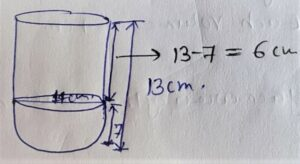Diameter = 14 cm

Radius = 14/2 = 7 cm

The inner surface area of the vessel = C.S.A. of Cylinder + C.S.A. of hemisphere

⇒ The inner surface area of the vessel = 2πrh + 2πr²

⇒ The inner surface area of the vessel = 2πr(h +r)

⇒ The inner surface area of the vessel = 2×22/7×7(6 +7)

⇒ The inner surface area of the vessel = 2×22×(13)

⇒ The inner surface area of the vessel = 572 cm²

Q.3 A toy is in the form a cone if radius 3.5 cm mounted in a hemisphere of same radius. The total height of the toy is 15.5 cm. Find the total surface area of the toy.

Given:

Total height = 15.5 cm

To Find:

The Total surface area of the toy =?

Solution:Slant height (l) = √(r²+h²)

⇒ l = √[(3.5)²+(12)²]

⇒ l = √[12.25+144]

⇒ l = √156.25

⇒ l = 12.5

Height of cone = Total Height – Radius of Hemisphere

⇒ h = 15.5 – 3.5

⇒ h = 12 cm

The total surface area of the toy = C.S.A. of cone + C.S.A. of hemisphere

⇒ The total surface area of the toy = πrl + 2πr²

⇒ The total surface area of the toy = πr( l + 2r)

⇒ The total surface area of the toy = 22/7 × 3.5 (12.5+7)

⇒ The total surface area of the toy = 22/7 × 3.5 (19.5)

⇒ The total surface area of the toy = 214.5 cm²

Q.4 A cubical block of side 7 cm is surmounted by a hemisphere. What is the greatest diameter the hemisphere can have ? Find the total surface area of the solid.

Given:

side = 7 cm

To Find:

Surface Area of Solid =?

Solution: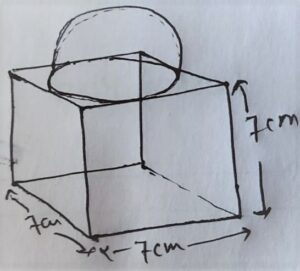Greatest diameter of hemisphere = Side if cube = 7cm.

r = 7/2

The surface area of the solid = T.S.A. of cub + C.S.A. of hemisphere – Base area of hemisphere

⇒ The surface area of the solid = 6a² + 2πr² – πr²

⇒ The surface area of the solid = 6a² + πr²

⇒ The surface area of the solid = 6×7×7 + 22/7 × 7/2×7/2

⇒ The surface area of the solid = 6×49 + 77/2

⇒ The surface area of the solid = 294 + 77/2

⇒ The surface area of the solid = (588+77)/2

⇒ The surface area of the solid = 665/2

⇒ The surface area of the solid = 332.5 cm²

Q.5. A hemispherical depression is cut out from one face of a cubical wooden block such that the diameter l of the hemisphere is equal to edge of the cube. determine the surface area of the remaining solid.

Solution: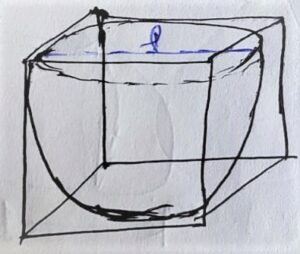Diameter = edge = l

Surface area of the remaining solid = T.S.A. of cube + C.S.A. of hemisphere – Base Area of hemisphere

⇒ Surface area of the remaining solid = 6a² + 2πr² – πr²

⇒ Surface area of the remaining solid = 6a² + 2πr² – πr²

⇒ Surface area of the remaining solid = 6a² + πr²

⇒ Surface area of the remaining solid = 6l² + π(l/2)²

⇒ Surface area of the remaining solid = 6l² + πl²/4

⇒ Surface area of the remaining solid = 6l² + πl²/4

⇒ Surface area of the remaining solid = (24l² + πl²)/4

⇒ Surface area of the remaining solid =1/4 ×l²(24 + π)

Q.6 A medicine capsule is in the shape of a cylinder with two hemispheres stuck to each of its ends (see figure 13.10).The length of the entire capsule is 14 mm and the diameter of the capsule is 5 mm. Find its surface area.

Solution:Diameter = 5 cm

r = 5/2

Height of Cylinder = 14 – (5/2 + 5/2) = 9 mm.

Surface Area of Capsule = C.S.A. of Cylinder + 2×C.S.A. of hemisphere

⇒ Surface Area of Capsule = 2πrh + 2×2πr²

⇒ Surface Area of Capsule = 2πrh + 4πr²

⇒ Surface Area of Capsule = 2πr(h + 2r)

⇒ Surface Area of Capsule = 2×22/7×5/2(9 + 2×5/2)

⇒ Surface Area of Capsule = 110/7(14)

⇒ Surface Area of Capsule = 220 mm²

Q.7 A tent is in the shape of a cylinder surmounted by a conical top. If the height and diameter of the cylindrical part are 2.1m and 4m respectively, and the slant height of the top is 2.8m, Find the area of the canvas used for making the tent. Also, find the cost of the canvas of the tent at the rate of Rs 500 per m².   ( Note that the base of the tent will not be covered with canvas )

Solution: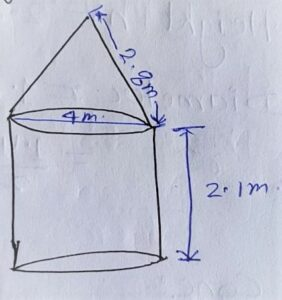Diameter of cylindrical part Height = 2.1

Diameter = 4m

Slant height of cone = l = 2.8 m

Radius of canvas used for making the rent = C.S.A. of cylinder + C.S.A. of cone

⇒ Radius of canvas used for making the rent = 2πrh + πrl

⇒ Radius of canvas used for making the rent = πr( 2h + l )

⇒ Radius of canvas used for making the rent = 22/7 × 2 × ( 2×2.1 + 2.8)

⇒ Radius of canvas used for making the rent = 22/7 × 2 × ( 4.2 + 2.8)

⇒ Radius of canvas used for making the rent = 22/7 × 2 × ( 7.0)

⇒ Radius of canvas used for making the rent = 44 m²

The cost of the canvas of tent =Rs 44×500

⇒The cost of the canvas of tent =R s 22000

Q.8 From a solid cylinder whose height is 2.4 cm and diameter 1.4 cm, a conical cavity of the same height and same diameter is hollowed out. Find the total surface area of the remaining solid to the nearest cm²

Solution: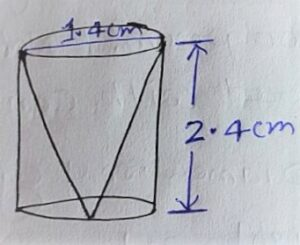Height(h) = 2.4 cm

Diameter = 1.4 cm

r= 1.4/2 =0.7 cm

Cone:

Slant height (l) = √(r²+h²)

⇒ Slant height (l) =√[(0.7)²+(2.4)²]

⇒ Slant height (l) =√[0.49+5.76]

⇒ Slant height (l) =√[6.25]

⇒ Slant height (l) =2.5

Total surface area of the remaining solid = C.S.A. of cone + C.S.A. of cylinder + upper area of cylinder

⇒ Total surface area of the remaining solid = πrl + 2πrh + πr²

⇒ Total surface area of the remaining solid = πr(l + 2h) + πr²

⇒ Total surface area of the remaining solid = 22/7×0.7(2.5 + 2×2.4) + 22/7 ×0.7×0.7

⇒ Total surface area of the remaining solid = 22/7×0.7(2.5 + 4.8) + 154/100

⇒ Total surface area of the remaining solid = 16.06+ 1.54

⇒ Total surface area of the remaining solid = 17.60≈18 cm²

Q.9 A wooden article was made by scooping out a hemisphere from each end of a solid cylinder, as shown in fig. 13.11. If the height of the cylinder is 10 cm, and its base is of radius 3.5 cm, Find the total surface area of the article.

Solution: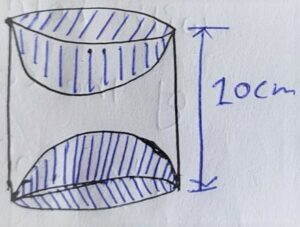Height (h) = 10 cm

r = 3.5 cm

Total Surface area of article = C.S.A. of cylinder + 2×C.S.A. of hemisphere

⇒ Total Surface area of article = 2πrh + 2× 2πr²

⇒ Total Surface area of article = 2πr(h + 2r)

⇒ Total Surface area of article = 2×22/7 ×3.5(10 + 2×3.5)

⇒ Total Surface area of article = 44/7 ×3.5(10 + 7.0)

⇒ Total Surface area of article = 44×5/7 ×3.5(17)

⇒ Total Surface area of article = 374 cm²